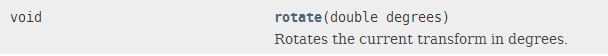# JavaFX旋转绘制的物体``````public void drawObject(GraphicsContext gc) {
gc.save();
gc.setGlobalAlpha(getAlpha());
Rotate r = new Rotate(getRotate(), getX() + getWidth() / 2, getY() + getHeight() / 2);
gc.setTransform(r.getMxx(), r.getMyx(), r.getMxy(), r.getMyy(), r.getTx(), r.getTy());
draw(gc);
gc.restore();
}``````

1.匿名说道：